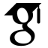Titel: New R-matrices for small quantum groups Sonstige Titel: Neue R-Matrizen für kleine Quantengruppen Sprache: Englisch Autor*in: Nett, Daniel Erscheinungsdatum: 2015 Tag der mündlichen Prüfung: 2015-03-16 Zusammenfassung: Quasitriangular Hopf algebras, i.e. Hopf algebras with an (universal) R-matrix, have braided categories of their modules. In particular, quasitriangular quantum groups yield ribbon categories, which have many interesting applications, especially in low- dimensional topology and topological quantum field theories.In this thesis we construct new universal R-matrices of the small quantum groups u_q(g) and extensions thereof. Here, g is always a finite-dimensional complex, simple Lie algebra and q is an l-th root of unity for an arbitrary integer l > 2. The first chapter of this thesis provides the necessary algebraic notions and gives the definition of the quantum groups in interest. Since in general the small quantum group is not quasitriangular, i.e. there exist no R-matrix, we consider certain extensions of the usual quantum group u_q(g), parametrized by a Lie theoretic input datum, namely a lattice Λ, containing the root lattice Λ_R and contained in the weight lattice Λ_W of g. This leads to the notion of u_q(g,Λ,Λ′) for the extension by Λ of the small quantum group uq(g,Λ′). Here, the lattice Λ′ ⊂ Λ_R determines a certain quotient in the construction of the quantum group.In the second chapter we review the ansatz R = R_0 Θ ̄ for R-matrices by Lusztig. This ansatz introduces a fixed element, the so-called quasi-R-matrix Θ, which is an intertwiner between the comultiplication ∆ and a new comultiplication ∆ ̄,obtained by conjugating with a certain antilinear involution. The free element R_0 is an intertwiner for ∆ ̄ and the opposed comultiplication ∆^{opp}. Eric Müller gives in his dissertation an ansatz and equations for the coefficients of R_0 in this ansatz and determines R-matrices of the form R = R_0 Θ ̄ for quadratic extensions of u_q(sl_n). In this thesis, we develop this ansatz further and get a new set of equations for the coefficients of R0, which we split in two different types. The first type of equations depends only on the fundamental group π_1 = Λ_W /Λ_R of the Lie algebra g and will be called group-equations. The second type depends on some sublattices of Λ and is very sensitive to different choices of l. This type of equations will be called diamond-equations.In Chapter 3 we determine the solutions to the group-equations. For the case of cyclic fundamental group π_1 = Z_N , N ∈ |N, (which is the case for all simple root systems but for D_{2m}, m ≥ 2), a theorem about certain idempotents of the group algebra C[Z_N ×Z_N ] is required. In order to prove this, we prove a main theorem about roots of unity first, which is of independent interest. The proof of this theorem is carried out along the lines of a new combinatorial principle, which to the best of our knowledge is introduced in this thesis. The solutions of the group-equations for the case of fundamental group π_1 = Z_2 × Z_2 are determined by a Maple-calculation which is documented in Appendix A.In Chapter 4 we determine all solutions of the diamond-equations from the set of the solutions of the group-equations. Again, we consider the case of cyclic fundamental group first and find that the existence of solutions then depends only on the funda- mental group π_1 of g and on the order l of the root of unity q. Depending on the data g, q, Λ (and fixed Λ′) we then determine in the main theorem all R-matrices obtained through Lusztig’s ansatz for different variants u_q(g,Λ,Λ′) of u_q(g). URL: https://ediss.sub.uni-hamburg.de/handle/ediss/6358 URN: urn:nbn:de:gbv:18-72654 Dokumenttyp: Dissertation Betreuer*in: Schweigert, Christoph (Prof. Dr.) Enthalten in den Sammlungen: Elektronische Dissertationen und Habilitationen

###### Dateien zu dieser Ressource:
Datei Beschreibung GrößeFormat
Dissertation.pdf608.71 kBAdobe PDF

Diese Publikation steht in elektronischer Form im Internet bereit und kann gelesen werden. Über den freien Zugang hinaus wurden durch die Urheberin / den Urheber keine weiteren Rechte eingeräumt. Nutzungshandlungen (wie zum Beispiel der Download, das Bearbeiten, das Weiterverbreiten) sind daher nur im Rahmen der gesetzlichen Erlaubnisse des Urheberrechtsgesetzes (UrhG) erlaubt. Dies gilt für die Publikation sowie für ihre einzelnen Bestandteile, soweit nichts Anderes ausgewiesen ist.

Info

#### Seitenansichten

25
Letzte Woche
Letzten Monat
geprüft am 17.04.2021

#### Download(s)

4
Letzte Woche
Letzten Monat
geprüft am 17.04.2021
WerkzeugePrüfe